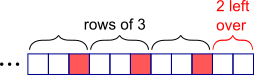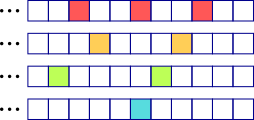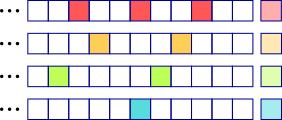#### You may also like### N000ughty Thoughts

How many noughts are at the end of these giant numbers?### DOTS Division

Take any pair of two digit numbers x=ab and y=cd where, without loss of generality, ab > cd . Form two 4 digit numbers r=abcd and s=cdab and calculate: {r^2 - s^2} /{x^2 - y^2}.### Mod 3

Prove that if a^2+b^2 is a multiple of 3 then both a and b are multiples of 3.

# Indivisible

##### Age 14 to 16 ShortChallenge Level

Lining up in rows of 3:Lining up in rows of each number:What comes next in each pattern?Next number is a multiple of 3, 4, 5, 6
Lowest common multiples of 3, 4, 5, 6: 60, 120, 180, etc.
Fewer than 100 students $\therefore$ there are 59 students.

This problem is taken from the UKMT Mathematical Challenges.
You can find more short problems, arranged by curriculum topic, in our short problems collection.